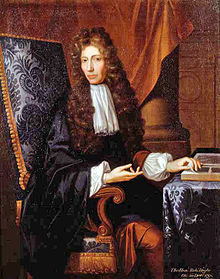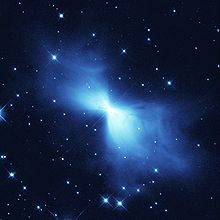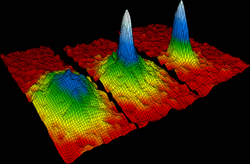# Absolute zero

Absolute zero

Absolute zero is the theoretical temperature at which entropy reaches its minimum value. The laws of thermodynamics state that absolute zero cannot be reached using only thermodynamic means. A system at absolute zero still possesses quantum mechanical zero-point energy, the energy of its ground state. The kinetic energy of the ground state cannot be removed. However, in the classical interpretation it is zero and the thermal energy of matter vanishes.

The zero point of any thermodynamic temperature scale, such as Kelvin or Rankine, is set at absolute zero. By international agreement, absolute zero is defined as 0K on the Kelvin scale and as −273.15 °C on the Celsius scale. This equates to −459.67 °F on the Fahrenheit scale and 0 °R on the Rankine scale. Scientists have achieved temperatures very close to absolute zero, where matter exhibits quantum effects such as superconductivity and superfluidity.

## HistoryRobert Boyle pioneered the idea of an absolute zero.

One of the first to discuss the possibility of an absolute minimal temperature was Robert Boyle. His 1665 New Experiments and Observations touching Cold, articulated the dispute known as the primum frigidum. The concept was well known among naturalists of the time. Some contended an absolute minimum temperature occurred within earth (as one of the four so-called 'elements'), others within water, others air, and some more recently within nitre. But all of them seemed to agree that, "There is some body or other that is of its own nature supremely cold and by participation of which all other bodies obtain that quality."

### Limit to the 'degree of cold'

The question whether there is a limit to the degree of cold possible, and, if so, where the zero must be placed, was first attacked by the French physicist Guillaume Amontons in 1702, in connection with his improvements in the air thermometer. In his instrument, temperatures were indicated by the height at which a column of mercury was sustained by a certain mass of air, the volume, or "spring", of which varied with the heat to which it was exposed. Amontons therefore argued that the zero of his thermometer would be that temperature at which the spring of the air in it was reduced to nothing. On the scale he used, the boiling-point of water was marked at +73 and the melting-point of ice at 51, so that the zero of his scale was equivalent to about −240 on the Celsius scale.[citation needed]

This close approximation to the modern value of −273.15 °C for the zero of the air-thermometer was further improved upon in 1779 by Johann Heinrich Lambert, who observed that −270 °C might be regarded as absolute cold.

Values of this order for the absolute zero were not, however, universally accepted about this period. Pierre-Simon Laplace and Antoine Lavoisier, in their 1780 treatise on heat, arrived at values ranging from 1,500 to 3,000 below the freezing-point of water, and thought that in any case it must be at least 600 below. John Dalton in his Chemical Philosophy gave ten calculations of this value, and finally adopted −3000 °C as the natural zero of temperature.

### Lord Kelvin's work

After J.P. Joule had determined the mechanical equivalent of heat, Lord Kelvin approached the question from an entirely different point of view, and in 1848 devised a scale of absolute temperature which was independent of the properties of any particular substance and was based solely on the fundamental laws of thermodynamics. It followed from the principles on which this scale was constructed that its zero was placed at −273.15 °C, at almost precisely the same point as the zero of the air-thermometer.

## Very low temperaturesThe rapid expansion of gases leaving the Boomerang Nebula causes the lowest observed temperature outside a laboratory.

The average temperature of the universe due to cosmic microwave background radiation today is 2.73 K.

Absolute zero cannot be achieved artificially, although it is possible to reach temperatures close to it through the use of cryocoolers. The use of laser cooling has produced temperatures less than a billionth of a kelvin. At very low temperatures in the vicinity of absolute zero, matter exhibits many unusual properties, including superconductivity, superfluidity, and Bose–Einstein condensation. To study such phenomena, scientists have worked to obtain even lower temperatures.

• The current world record was set in 1999 at 100 picokelvins (pK), or 0.000 000 000 1 of a Kelvin, by cooling the nuclear spins in a piece of rhodium metal.
• In February 2003, the Boomerang Nebula was observed to have been releasing gases at a speed of 500,000 km/h (over 300,000 mph) for the last 1,500 years. This has cooled it down to approximately 1 K, as deduced by astronomical observation, which is the lowest natural temperature ever recorded.
• In May 2006, the Institute of Quantum Optics at the University of Hanover gave details of technologies and benefits of femto-kelvin research in space.

## Thermodynamics near absolute zero

At temperatures near 0 K, nearly all molecular motion ceases and, when entropy = S, ΔS = 0 for any adiabatic process. Pure substances can (ideally) form perfect crystals as T → 0. Max Planck's strong form of the third law of thermodynamics states the entropy of a perfect crystal vanishes at absolute zero. The original Nernst heat theorem makes the weaker and less controversial claim that the entropy change for any isothermal process approaches zero as T → 0:$\lim_{T \to 0} \Delta S = 0$

The implication is that the entropy of a perfect crystal simply approaches a constant value.

The Nernst postulate identifies the isotherm T = 0 as coincident with the adiabat S = 0, although other isotherms and adiabats are distinct. As no two adiabats intersect, no other adiabat can intersect the T = 0 isotherm. Consequently no adiabatic process initiated at nonzero temperature can lead to zero temperature. (≈ Callen, pp. 189–190)

An even stronger assertion is that It is impossible by any procedure to reduce the temperature of a system to zero in a finite number of operations. (≈ Guggenheim, p. 157)

A perfect crystal is one in which the internal lattice structure extends uninterrupted in all directions. The perfect order can be represented by translational symmetry along three (not usually orthogonal) axes. Every lattice element of the structure is in its proper place, whether it is a single atom or a molecular grouping. For substances which have two (or more) stable crystalline forms, such as diamond and graphite for carbon, there is a kind of "chemical degeneracy". The question remains whether both can have zero entropy at T = 0 even though each is perfectly ordered.

Perfect crystals never occur in practice; imperfections, and even entire amorphous materials, simply get "frozen in" at low temperatures, so transitions to more stable states do not occur.

Using the Debye model, the specific heat and entropy of a pure crystal are proportional to T 3, while the enthalpy and chemical potential are proportional to T 4. (Guggenheim, p. 111) These quantities drop toward their T = 0 limiting values and approach with zero slopes. For the specific heats at least, the limiting value itself is definitely zero, as borne out by experiments to below 10 K. Even the less detailed Einstein model shows this curious drop in specific heats. In fact, all specific heats vanish at absolute zero, not just those of crystals. Likewise for the coefficient of thermal expansion. Maxwell's relations show that various other quantities also vanish. These phenomena were unanticipated.

Since the relation between changes in Gibbs free energy (G), the enthalpy (H) and the entropy is$\Delta G = \Delta H - T \Delta S \,$

thus, as T decreases, ΔG and ΔH approach each other (so long as ΔS is bounded). Experimentally, it is found that all spontaneous processes (including chemical reactions) result in a decrease in G as they proceed toward equilbrium. If ΔS and/or T are small, the condition ΔG < 0 may imply that ΔH < 0, which would indicate an exothermic reaction. However, this is not required; endothermic reactions can proceed spontaneously if the TΔS term is large enough.

Moreover, the slopes of the derivatives of ΔG and ΔH converge and are equal to zero at T = 0. This ensures that ΔG and ΔH are nearly the same over a considerable range of temperatures and justifies the approximate empirical Principle of Thomsen and Berthelot, which states that the equilibrium state to which a system proceeds is the one which evolves the greatest amount of heat, i.e. an actual process is the most exothermic one. (Callen, pp. 186–187)

One model that estimates the properties of an electron gas at absolute zero in metals is the Fermi gas. The electrons, being Fermions, have to be in different quantum states, which leads the electrons to get very high typical velocities, even at absolute zero. The maximum energy that an electrons can have at absolute zero is called the Fermi energy. The Fermi temperature is defined as this maximum energy divided by Boltzmann's constant, and is of the order of 80,000 K for typical electron densities found in metals. For temperatures significantly below the Fermi temperature, the electrons behave in almost the same way as at absolute zero. This explains the failure of the classical equipartition theorem for metals that eluded classical physicists in the late 19th century.

## Relation with Bose–Einstein condensatesVelocity-distribution data of a gas of rubidium atoms at a temperature within a few billionths of a degree above absolute zero. Left: just before the appearance of a Bose–Einstein condensate. Center: just after the appearance of the condensate. Right: after further evaporation, leaving a sample of nearly pure condensate.

A Bose–Einstein condensate (BEC) is a state of matter of a dilute gas of weakly interacting bosons confined in an external potential and cooled to temperatures very near to absolute zero. Under such conditions, a large fraction of the bosons occupy the lowest quantum state of the external potential, at which point quantum effects become apparent on a macroscopic scale.

This state of matter was first predicted by Satyendra Nath Bose and Albert Einstein in 1924–25. Bose first sent a paper to Einstein on the quantum statistics of light quanta (now called photons). Einstein was impressed, translated the paper himself from English to German and submitted it for Bose to the Zeitschrift für Physik which published it. Einstein then extended Bose's ideas to material particles (or matter) in two other papers.

Seventy years later, the first gaseous condensate was produced by Eric Cornell and Carl Wieman in 1995 at the University of Colorado at Boulder NIST-JILA lab, using a gas of rubidium atoms cooled to 170 nanokelvin (nK) (1.7×10−7 K).

A record cold temperature of 450 ±80 pK in a Bose–Einstein condensate (BEC) of sodium atoms was achieved in 2003 by researchers at MIT. It's noteworthy that this record's peak emittance black-body wavelength of 6,400 kilometers is roughly the radius of Earth.

## Absolute temperature scales

Absolute, or thermodynamic, temperature is conventionally measured in kelvins (Celsius-scaled increments) and in the Rankine scale (Fahrenheit-scaled increments) with increasing rarity. Absolute temperature measurement is uniquely determined by a multiplicative constant which specifies the size of the "degree", so the ratios of two absolute temperatures, T2/T1, are the same in all scales. The most transparent definition of this standard comes from the Maxwell–Boltzmann distribution. It can also be found in Fermi–Dirac statistics (for particles of half-integer spin) and Bose–Einstein statistics (for particles of integer spin). All of these define the relative numbers of particles in a system as decreasing exponential functions of energy (at the particle level) over kT, with k representing the Boltzmann constant and T representing the temperature observed at the macroscopic level.

## Negative temperatures

Temperatures that are expressed as negative numbers on the familiar Celsius or Fahrenheit scales are simply colder than the zero points of those scales. Certain systems can achieve truly negative temperatures; that is, their thermodynamic temperature (expressed in kelvin) can be of a negative quantity. A system with a truly negative temperature is not colder than absolute zero. Rather, a system with a negative temperature is hotter than any system with a positive temperature in the sense that if a negative-temperature system and a positive-temperature system come in contact, heat will flow from the negative- to the positive-temperature system.

Most familiar systems cannot achieve negative temperatures because adding energy always increases their entropy. However, some systems have a maximum amount of energy that they can hold, and as they approach that maximum energy their entropy actually begins to decrease. Because temperature is defined by the relationship between energy and entropy, such a system's temperature becomes negative, even though energy is being added. As a result, the Boltzmann factor for states of systems at negative temperature increases rather than decreases with increasing state energy. Therefore no complete system, i.e. including the electromagnetic modes, can have negative temperatures, since there is no highest energy state, so that the sum of the probabilities of the states would diverge for negative temperatures. However, for quasi-equilibrium systems (e.g. spins out of equilibrium with the electromagnetic field) this argument does not apply, and negative effective temperatures are attainable.

Wikimedia Foundation. 2010.

### Look at other dictionaries:

• Absolute zero — Absolute Ab so*lute, a. [L. absolutus, p. p. of absolvere: cf. F. absolu. See {Absolve}.] 1. Loosed from any limitation or condition; uncontrolled; unrestricted; unconditional; as, absolute authority, monarchy, sovereignty, an absolute promise or …   The Collaborative International Dictionary of English

• Absolute zero — Zero e ro, n.; pl. {Zeros}or {Zeroes}. [F. z[ e]ro, from Ar. [,c]afrun, [,c]ifrun, empty, a cipher. Cf. {Cipher}.] [1913 Webster] 1. (Arith.) A cipher; nothing; naught. [1913 Webster] 2. The point from which the graduation of a scale, as of a… …   The Collaborative International Dictionary of English

• Absolute Zero — Éditeur Eidos Interactive Développeur Domark Dat …   Wikipédia en Français

• absolute zero — the idea dates back to 1702 and its general value was guessed to within a few degrees soon thereafter, but not precisely discovered until Lord Kelvin s work in 1848. It was known by many names, e.g. infinite cold, absolute cold, natural zero of… …   Etymology dictionary

• absolute zero — ► NOUN ▪ the lowest temperature theoretically possible (zero kelvins, 273.15°C) …   English terms dictionary

• absolute zero — n a theoretical temperature characterized by complete absence of heat and equivalent to exactly 273.15°C or 459.67°F * * * the lowest possible temperature, designated as 0 on the Kelvin or Rankine scale; by definition this is equivalent to… …   Medical dictionary

• absolute zero — n [U] the lowest temperature that is believed to be possible …   Dictionary of contemporary English

• absolute zero — noun uncount 273ºC or 460ºF, the lowest temperature that is possible in theory …   Usage of the words and phrases in modern English

• absolute zero — n. the temperature at which matter has no heat and its molecules are completely still; theoretically, the lowest possible temperature: equal to about 273.16°C or about 459.69°F or 0°K …   English World dictionary

• absolute zero — the temperature of 273.16°C ( 459.69°F), the hypothetical point at which all molecular activity ceases. * * * Temperature at which a thermodynamic system (see thermodynamics) has the lowest energy, 0 kelvin (K). It corresponds to 459.67°F (… …   Universalium